• [Machine Learning] 梯度下降法的三种形式BGD、SGD以及MBGD
• 2018年03月24日
• 网络收集

在应用机器学习算法时，我们通常采用梯度下降法来对采用的算法进行训练。其实，常用的梯度下降法还具体包含有三种不同的形式，它们也各自有着不同的优缺点。

下面我们以线性回归算法来对三种梯度下降法进行比较。

一般线性回归函数的假设函数为：

$h_{\theta}=\sum_{j=0}^{n}\theta_{j}x_{j}$

对应的能量函数（损失函数）形式为：

$J_{train}(\theta)=1/(2m)\sum_{i=1}^{m}(h_{\theta}(x^{(i)})-y^{(i)})^{2}$

下图为一个二维参数（$\theta_{0}$和$\theta_{1}$）组对应能量函数的可视化图：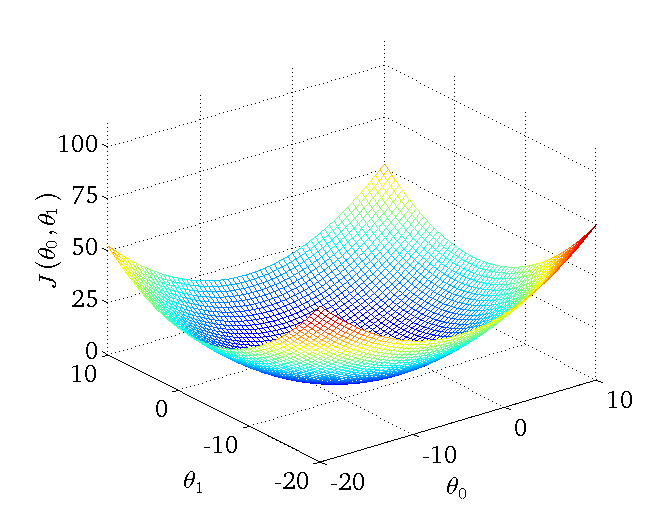### 1. 批量梯度下降法BGD

(1) 对上述的能量函数求偏导：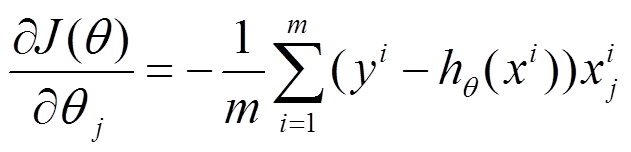(2) 由于是最小化风险函数，所以按照每个参数$\theta$的梯度负方向来更新每个$\theta$：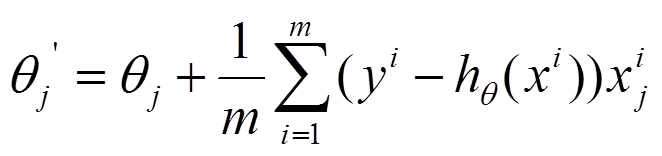具体的伪代码形式为：

repeat {（for every j=0, ... , n）

}

从上面公式可以注意到，它得到的是一个全局最优解，但是每迭代一步，都要用到训练集所有的数据，如果样本数目$m$很大，那么可想而知这种方法的迭代速度！所以，这就引入了另外一种方法，随机梯度下降。

从迭代的次数上来看，BGD迭代的次数相对较少。其迭代的收敛曲线示意图可以表示如下：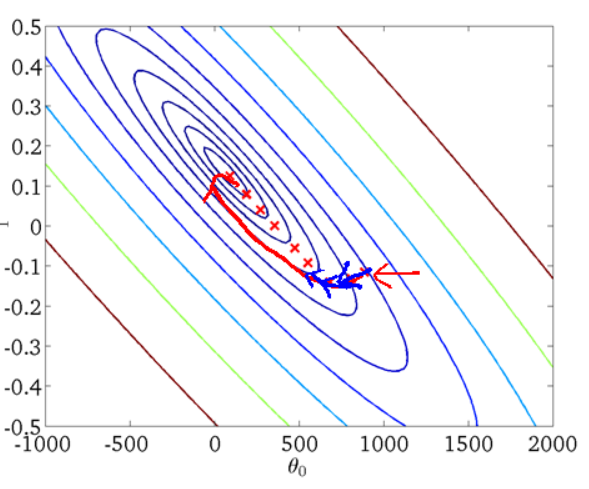### 2. 随机梯度下降法SGD

将上面的能量函数写为如下形式：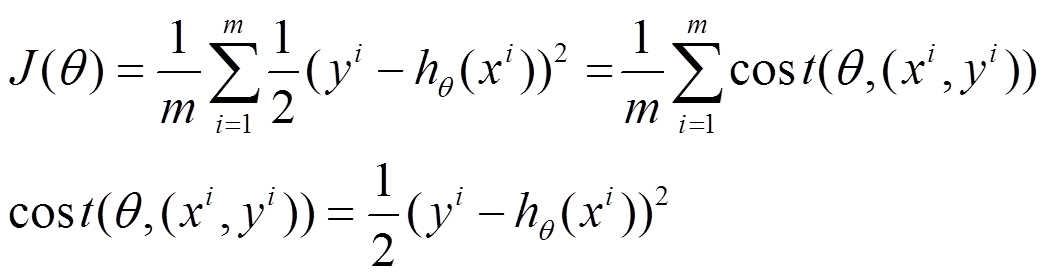利用每个样本的损失函数对$\theta$求偏导得到对应的梯度，来更新$\theta$：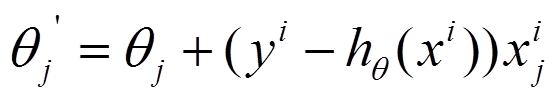具体的伪代码形式为：

1. Randomly shuffle dataset；

2.  repeat {

for i=1, ... , $m${(for j=0, ... , $n$)

}

}

随机梯度下降是通过每个样本来迭代更新一次，如果样本量很大的情况（例如几十万），那么可能只用其中几万条或者几千条的样本，就已经将theta迭代到最优解了，对比上面的批量梯度下降，迭代一次需要用到十几万训练样本，一次迭代不可能最优，如果迭代10次的话就需要遍历训练样本10次。但是，SGD伴随的一个问题是噪音较BGD要多，使得SGD并不是每次迭代都向着整体最优化方向。

从迭代的次数上来看，SGD迭代的次数较多，在解空间的搜索过程看起来很盲目。其迭代的收敛曲线示意图可以表示如下：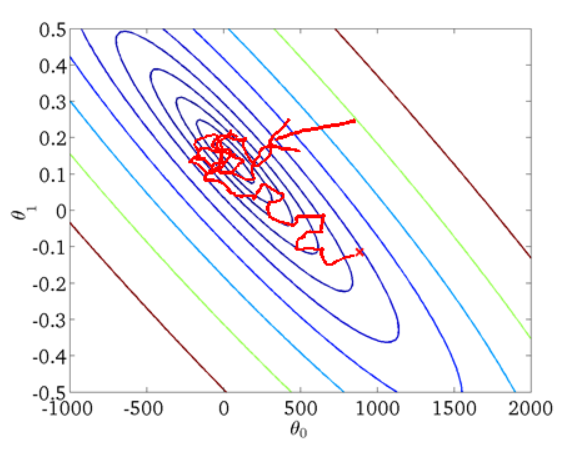### 3. 小批量梯度下降法MBGD

MBGD在每次更新参数时使用b个样本（b一般为10），其具体的伪代码形式为：

Say b=10, m=1000.

Repeat {

for i=1, 11, 21, 31, ... , 991{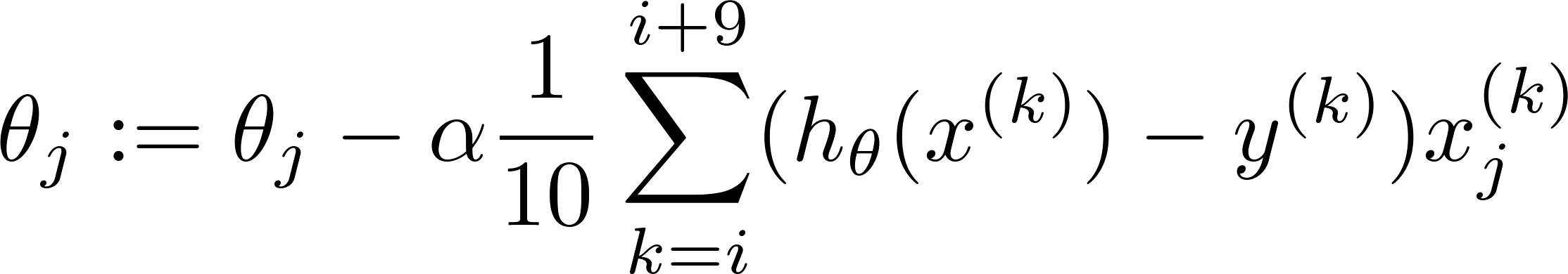(for every j=0, ... , $n$)

}

}

### 4. 总结

Batch gradient descent: Use all examples in each iteration；

Stochastic gradient descent: Use 1 example in each iteration；

Mini-batch gradient descent: Use b examples in each iteration.